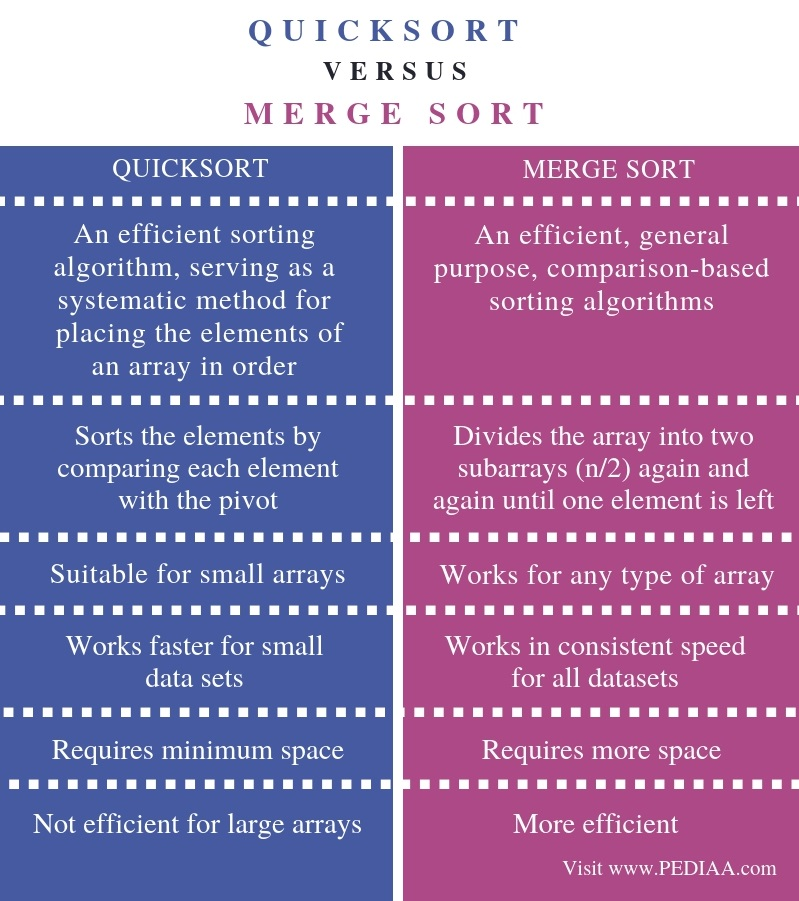# What is the Difference Between Quicksort and Merge Sort

The main difference between quicksort and merge sort is that the quicksort sorts the elements by comparing each element with an element called a pivot while merge sort divides the array into two subarrays again and again until one element is left.

Sorting is the method of arranging data in a particular order. When arranging the data, it is possible to consider the numerical or lexicographical order. Sorting helps to search and access data elements faster and quicker. There are various sorting algorithms and quicksort and merge sort are two of them.

### Key Areas Covered

1. What is Quicksort
– Definition, Functionality
2. What is Merge Sort
– Definition, Functionality
3. What is the Difference Between Quicksort and Merge Sort
– Comparison of Key differences

### Key Terms

Algorithm, Array, Merge Sort, Quicksort## What is Quicksort

Quicksort is an internal algorithm that uses ‘divide and conquer technique’. It is also called a partition exchange sort. It uses a key element called pivot for comparing and partitioning the elements in the array. The items with a lesser value than the pivot go to the left side of the pivot while items with a greater value than the pivot go to the right side of the pivot. The left section is called the left partition, and the right section is called the right partition.Figure 1: Quicksort

Refer to the below example.

36  34  43  11  15 20 28 45  27  32

Consider 32 as the pivot and consider 36 and 27. The conditions 36 < pivot, 27 > pivot are false. Therefore, we can swap these two values. Now the list is as follows.

27  34  43  11  15  20  28  45  36  32

Consider the values 34 and 45. When considering 34 < pivot, the condition is false. Similarly, 45 > pivot condition is true. Now, we can move from 45 to 28. Let’s consider 34 and 28. 34 < pivot is false and 28 > pivot is false. Therefore, we can swap 34 and 28.

27 28  43  11  15  20  34  45  36  32

Consider 43 and 20. 43 < pivot is false. 20 > pivot is false. Therefore, we can swap the two numbers. Now the list is as follows.

27  28  20  11  15  43  34  45 36  32

Now consider 11 and 15. 11 < pivot is true. We can consider 15. It is less than 32. It is the overlapping point, and we can place 32 as follows.

27  28  20  11  15  32  43  34  45  36

Now the numbers in the left side of the pivot are smaller than the pivot, and the right side of the pivot are greater than the pivot. We can apply quicksort to the left and right partitions to sort the entire list.

## What is Merge Sort

Merge sort is an external algorithm that uses ‘divide and conquer technique’. It splits the array into two sections. It sorts each array and combines them together to form the sorted array. Merge sort requires additional storage to sort the auxiliary array.

Consider the following example.Figure 2: Merge Sort

We can divide the array into two sections. Now there are two arrays as follows.

38  27  43  3         9  82  10

Consider 38  27  43  3. We can divide it into two arrays again. They are 38  27  and 43 3.  38 27 divides into 38 and 27 while 43 3 divides into 43 and 3.  Sorting 38 and 27 gives 27   38. Sorting 43 3 gives 3 43.  Now it is possible to combine 27  38  and 3  43.  After sorting them, we get an array as 3  27 38  43.

Similarly, consider 9 82 10. We can divide it into two arrays again. They are 9 82 and 10. 9 82 divides into 9 and 82. Moreover, there is number 10 in the other array. 9 and 82 sort as 9  82. Thus, this array and array with value 10 combines and provides 9  10 and 82.

Finally, 3  27  38  43 and 9  10  82 combine to provide the sorted array.

## Difference Between Quicksort and Merge Sort

### Definition

Quicksort is an efficient sorting algorithm, serving as a systematic method for placing the elements of an array in order. In contrast, merge sort is an efficient, general purpose, comparison-based sorting algorithms. Thus, this is the fundamental difference between quicksort and merge sort.

### Functionality

Aboveall, the functionality is the main difference between quicksort and merge sort. Quicksort sorts the elements by comparing each element with the pivot while merge sort divides the array into two subarrays (n/2) again and again until one element is left.

### Application

Also, while quicksort is suitable for small arrays, merge sort works for any type of array.

### Speed

Another difference between quicksort and merge sort is that the quicksort works faster for small data sets while merge sort works in consistent speed for all datasets.

### Space Requirement

Moreover, the space requirement is also an important difference between quicksort and merge sort. Quicksort requires minimum space compared to merge sort.

### Efficiency

Furthermore, quicksort is not efficient for large arrays, but merge sort is more efficient than quicksort. Hence, this is another difference between quicksort and merge sort.

### Conclusion

In summary, the main difference between quicksort and merge sort is that the quicksort sorts the elements by comparing each element with an element called a pivot while the merge sort divides the array into two subarrays again and again until one element is left.

##### Reference:

1. Quicksort Algorithm| Part 2, Education 4u, 15 Mar. 2018, Available here.
2. Merge Sort Example, Education 4u, 15 Mar. 2018, Available here.

##### Image Courtesy:

1. “Quicksort-diagram” By Znupi – Own work (Public Domain) via Commons Wikimedia
2. “Merge sort algorithm diagram” By Vineet Kumar at English Wikipedia – Transferred from en.wikipedia to Commons by Eric Bauman using CommonsHelper (Public Domain) via Commons Wikimedia